#There are 366 different Starters of The Day, many to choose from. You will find below some starters on the topic of Mixed. A lesson starter does not have to be on the same topic as the main part of the lesson or the topic of the previous lesson. It is often very useful to revise or explore other concepts by using a starter based on a totally different area of Mathematics.

Main Page

### Mixed Starters: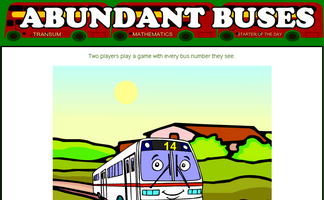A game based around the concept of factors and abundant numbers.Make more of analogies to help remember mathematical concepts.Estimate or calculate then put the large numbers in order of size.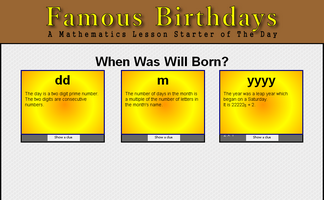Work out the date Will was born by answering some number questions.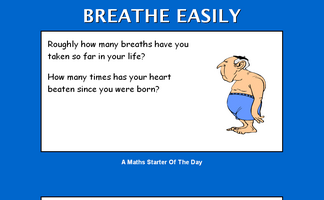How many breaths have you taken in your lifetime?How many palindromic numbers can you find?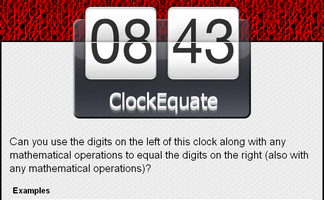Can you use the digits on the left of this clock along with any mathematical operations to equal the digits on the right?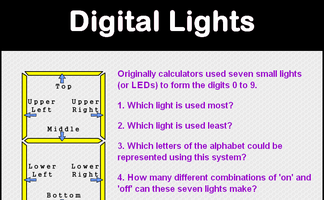Questions about the Small LEDs used to make up the digits on a calculator display.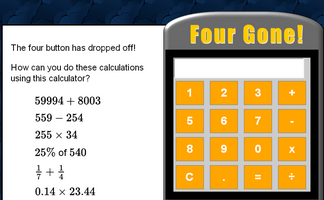An activity involving a broken calculator which is missing the four button. Can you evaluate the given expressions without using the four?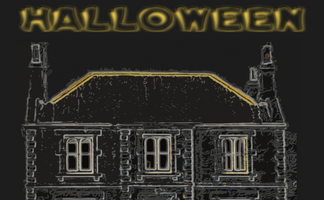Five problems with a Halloween theme.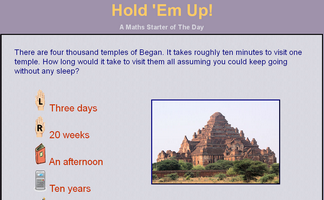Everyone in the class holds up an item to show which is the correct answer to the multiple choice question.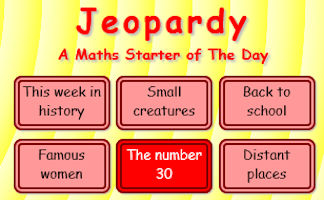Loosely based on the famous television show, how many questions cn you come up with for a given answer?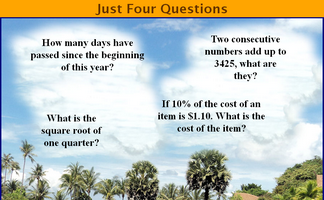Four mathematical questions to think about at the beginning of the lesson.The 31st of December is the last day of the year. What mathematical lasts do you know?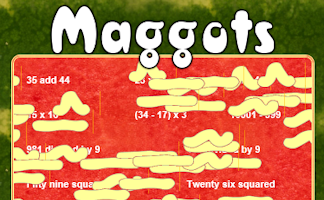Do as many of the calculations as possible before the maggots infest!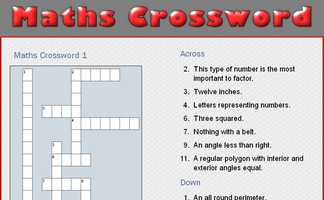A small crossword puzzle containing mathematical words.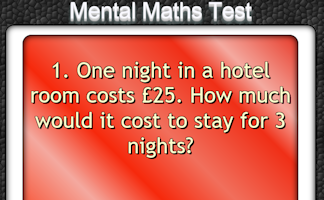The starter is a PowerPoint file containing a twenty question mental arithmetic test. It will advance from one question to the next automatically.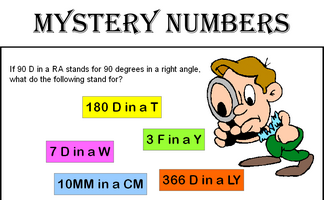Can you recognise the mystery numbers from the clues?Can you solve this paradox by agreeing whether the statements are right or wrong?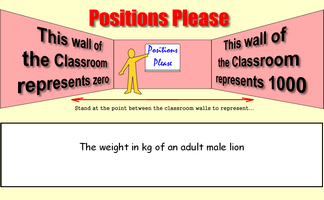Stand at the point between the classroom walls to represent a given number.It is called Refreshing Revision because every time you refresh the page you get different revision questions.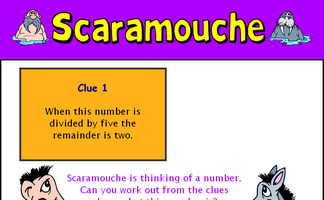Can you work out from the five clues given what the mystery number is?Six calculations to perform without a calculator.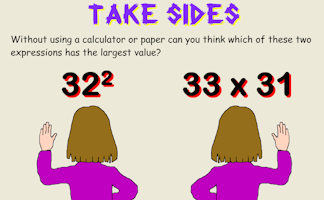Put up your right hand or left hand depending on the expressions that appears.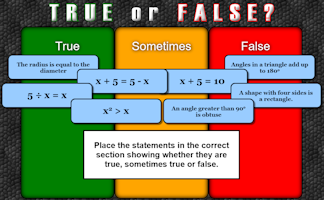An activity designed to address common misconceptions.Create your own mathematical word search for the whole class to enjoy.

### Search

The activity you are looking for may have been classified in a different way from the way you were expecting. You can search the whole of Transum Maths by using the box below.

Have today's Starter of the Day as your default homepage. Copy the URL below then select
Tools > Internet Options (Internet Explorer) then paste the URL into the homepage field.

Set as your homepage (if you are using Internet Explorer)

Do you have any comments? It is always useful to receive feedback and helps make this free resource even more useful for those learning Mathematics anywhere in the world. Click here to enter your comments.For All:

©1997-2019 WWW.TRANSUM.ORG